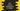# Dart program to convert hexadecimal to integer### Dart program to convert hexadecimal to integer:

In this post, we will learn how to convert one hexadecimal value to an integer value in dart. Dart integer class provides one parse method that we can use to convert a hexadecimal value to integer.

This post will show you how to convert hexadecimal to integer value using parse.

### Definition of parse:

The parse method is defined as below:

``int parse(String sourceValue {, int radix})``

This method is used to convert one string value to integer. The radix value can be anything from 2 to 36. For hexadecimal values, we can use 16 as radix. This will convert hexadecimal to integer.

### Example of parsing hexadecimal values:

Let’s consider the below example:

``````void main() {
String string_1 = "AA";
String string_2 = "3E8";
String string_3 = "EEE";

}``````

It prints the below output:

``````string_1 170
string_2 1000
string_3 3822``````

We can also use 0x at the start of the hexadecimal values. In that case, we don’t have to pass the radix value.

``````void main() {
String string_1 = "0xAA";
String string_2 = "0x3E8";
String string_3 = "0xEEE";

print("string_1 \${int.parse(string_1)}");
print("string_2 \${int.parse(string_2)}");
print("string_3 \${int.parse(string_3)}");
}``````

It prints the same output.